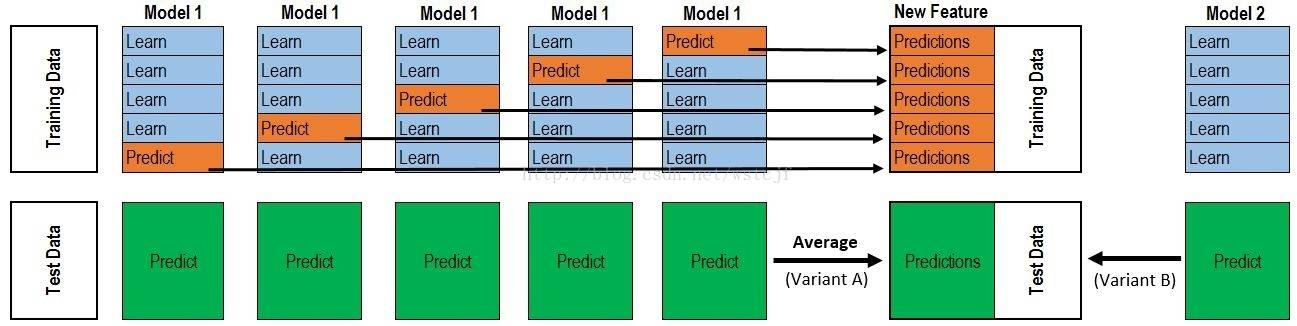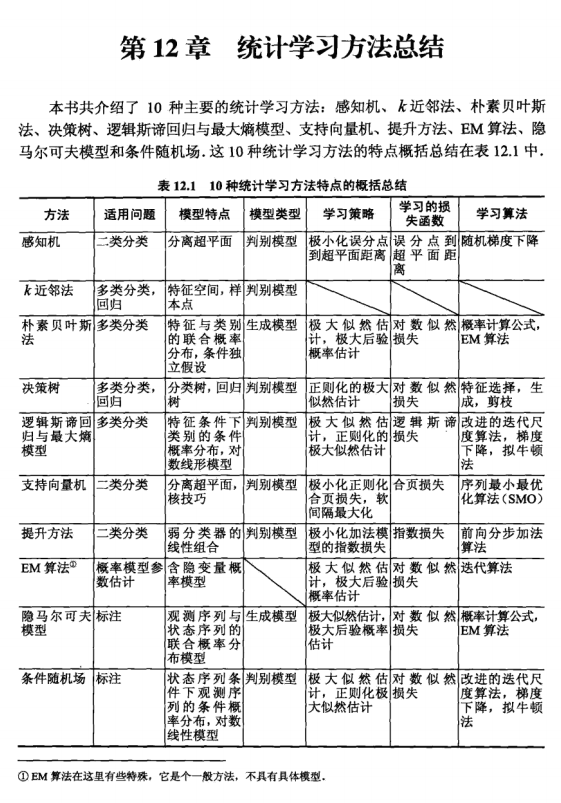# 模型融合Blending 和 Stacking

30 篇文章 0 订阅

### 前言

• 什么是融合？
• 几种方式融合
• 基本的模型融合组合及适用场景、优缺点等

### 什么是融合？

• Blending
• Stacking

• 准确性
要求该模型的性能不能太差
• 差异性
选择模型一般是多个，要求这多个模型之间有差异，有差异才能通过融合模型发挥他们的优势。

### Blending融合方式

##### Uniform Blending (均匀融合)

G ( x ) = s i g n ( ∑ t = 1 T 1 ∗ g t ( x ) ) G(x) = sign(\sum^{T}_{t=1}1*g_t(x)) \\

##### Linear Blending (线性融合)

G ( x ) = s i g n ( ∑ t = 1 T a t g t ( x ) ) a t ≥ 0 G(x) = sign(\sum^{T}_{t=1}a_tg_t(x)) \\ a_t \ge 0

best_p = 0
best_score = 0
for p in range(0,1,0.05):
b_score = model(lgbmodel,xgbmodel,p)
if b_score > best_score :
best_score = b_score
best_p = p


lgb和xgb均用了3个不同种子的5折融合，相当于最后一共融合了30个模型的预测结果，这样的操作使最终线上得分突破了0.5。

### Stacking融合方式

Blending方式各个分类器直接相对都是独立的，Stacking则有点像组合方式，每一个层都是一个模型，下一层模型利用上一层模型的输出来得到结果作为下一层输入，但Stacking算法分为2层，第一层是用不同的算法形成T个弱分类器，同时产生一个与原数据集大小相同的新数据集，利用这个新数据集和一个新算法构成第二层的分类器。

• (1) 先将训练集D拆成k个大小相似但互不相交的子集 D 1 , D 2 , … , D k D_1,D_2,…,D_k
• (2) 令 D j ′ = D − D j D_j'= D - D_j ，在 D j ′ D_j' 上训练一个弱学习器 L j L_j 。将 D j D_j 作为测试集，获得 L j L_j D j D_j 上的输出 D j ′ D_j' ，这里 j = 1... n j=1...n ，也就是说有1个模型对应k个弱学习器；
• (3) 步骤2可以得到k个弱学习器以及k个相应的输出 D j ′ D_j' ，这个k个输出加上原本的类标签构成新的训练集 D n D_n 这里的输入特征怎么就变成一个呢？
• (4) 在 D n D_n 训练次学习器 L L L L 即为最后的学习器。• Training Data ：表示是测试集，完全的测试集，这个测试集会被分割成K份，其中一份作为验证集，其他用来训练模型。
• 一个模型因为数据集划分的不同，要训练成k次，每一个得到一个弱学习器，模型类型是一样的，但参数有可能会不同，毕竟训练集、测试集不同
• Test Data：测试集，这个不划分K份，那么一个模型k个弱学习器，预测是结果是一个样本有k个输出结果
• 一个测试集样本有k个输出怎么作为下一层模型的输入呢？答案是上图绿色框框部分的求平均值，k个值平均后就变成了一行数据，这一行数据作为特征，用来第二层的输入。

• 训练集：每一个样本有m个输出，假如样本数是1000，m=3，那么输出就是 1000 ∗ 3 1000*3 的矩阵
• 测试集：每一个样本有m个输出，假如样本数是100，m=3，那么输出就是 100 ∗ 3 100*3 的矩阵，跟测试集一样的矩阵大小是因为有取平均值。

• 1、把训练集分为不交叉的五份。我们标记为train1到train5。
• 2、选一个基模型model_1，用train2、train3、train4、train5作为训练集，train1作为验证集，这样训练参数得到一个模型model_1_1，并用这个模型预测train1，这样train1也得到了pred1。
• 3、依次用train2作为验证集，其他四份作为训练集，得到model_1_2，这样一个基模型在train1-train5上有5个模型，同时，train1到train5都有预测值：pred1 - pred5；
• 4、再选基模型 mode_2 重复第2、3步骤。
• 5、考虑test数据集，每一个基模型对与test都有一次预测，但基模型在train上有5个模型，因此test的预测结果也有5次，会对这5次结果求平均值用于作为下一层输入

• 一般第二层采用LR模型，也叫做meta-model 元模型。
• 因为我们有3个基模型，所以输入的大小是n3，n表示样本大小，3表示基模型数量，一个样本被一个基模型预测一次，并且有一个预测值，这样输入就是n3。
• 输出就是样本的标签值。
• 利用meta-model 对上述新数据进行建模预测，预测出来的数据就是提交的最终数据。

• 第一层训练中只说了三种算法形成三份训练集Predictions作为第二层的特征，其实三种特征有点少，容易overfitting，尽量多用一些算法，每种算法也可以根据hyperopt的搜索参数对应不同的模型，这样就会有很多模型产生，也就是会形成多份“训练集Predictions”以及多份“测试集Predictions”，这样在第二层建立的模型及预测的结果相对会好一些。

stacking融合，加入NN和逻辑回归增强泛化能力。

### 基本的模型融合组合及适用场景、优缺点等### 举例说明

XGBClassifier、RFClassifier 作为基模型，采用 LogisticRegressionCV 作为次模型。

from sklearn import datasets
from sklearn import model_selection
from sklearn.linear_model import LogisticRegression
from sklearn.neighbors import KNeighborsClassifier
from sklearn.naive_bayes import GaussianNB
from sklearn.ensemble import RandomForestClassifier
from mlxtend.classifier import StackingCVClassifier
import numpy as np
import warnings

warnings.simplefilter('ignore')

X, y = iris.data[:, 1:3], iris.target

RANDOM_SEED = 42

clf1 = KNeighborsClassifier(n_neighbors=1)
clf2 = RandomForestClassifier(random_state=RANDOM_SEED)
clf3 = GaussianNB()
lr = LogisticRegression()

# Starting from v0.16.0, StackingCVRegressor supports
# random_state to get deterministic result.
sclf = StackingCVClassifier(classifiers=[clf1, clf2, clf3],meta_classifier=lr,use_probas=True, cv=5)

print('3-fold cross validation:\n')

for clf, label in zip([clf1, clf2, clf3, sclf],
['KNN',
'Random Forest',
'Naive Bayes',
'StackingClassifier']):

scores = model_selection.cross_val_score(clf, X, y,cv=3, scoring='accuracy')
print("Accuracy: %0.2f (+/- %0.2f) [%s]" % (scores.mean(), scores.std(), label))



3-fold cross validation:

Accuracy: 0.91 (+/- 0.01) [KNN]
Accuracy: 0.90 (+/- 0.03) [Random Forest]
Accuracy: 0.92 (+/- 0.03) [Naive Bayes]
Accuracy: 0.95 (+/- 0.03) [StackingClassifier]


。大家就算是来尝试一下这个模型融合的流程。

### stacking 回归

from mlxtend.regressor import StackingCVRegressor
from mlxtend.data import boston_housing_data
from sklearn.linear_model import LinearRegression, Ridge, Lasso
from sklearn.svm import SVR
from sklearn.model_selection import train_test_split, GridSearchCV, cross_val_score
from sklearn.metrics import mean_squared_error
import numpy as np
import matplotlib.pyplot as plt

x, y = boston_housing_data()
x = x[:100]
y = y[:100]
# 划分数据集
x_train, x_test, y_train, y_test = train_test_split(x, y, test_size=0.2)
# 初始化基模型
lr = LinearRegression()
svr_lin = SVR(kernel='linear', gamma='auto')
ridge = Ridge(random_state=2019,)
lasso =Lasso()
models = [lr, svr_lin, ridge, lasso]

params = {'lasso__alpha': [0.1, 1.0, 10.0],
'ridge__alpha': [0.1, 1.0, 10.0]}

sclf = StackingCVRegressor(regressors=models, meta_regressor=ridge)
grid = GridSearchCV(estimator=sclf, param_grid=params, cv=5, refit=True)
grid.fit(x_train, y_train)
print(grid.best_score_, grid.best_params_)



StackingCVRegressor
Stacking demo

### 总结

Blending 主要在优化variance（即模型的鲁棒性），Stacking主要在优化bias（即模型的精确性）。这是从另一个角度来看这个问题。

### 参考博客

03-26
11-28142401-074万+
01-125万+
06-018205
08-301万+
05-05867
05-1143
02-031754
03-297785
08-141万+
08-118043
02-271472
08-275万+
08-271773
08-026826
08-303万+
04-01441
11-195636
05-11523点击重新获取扫码支付余额充值